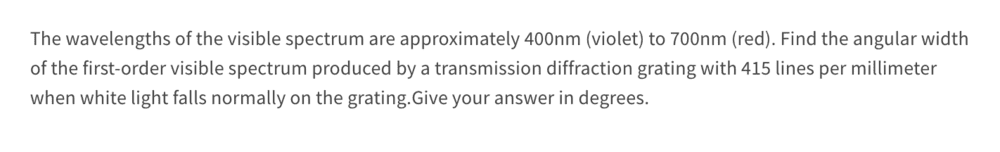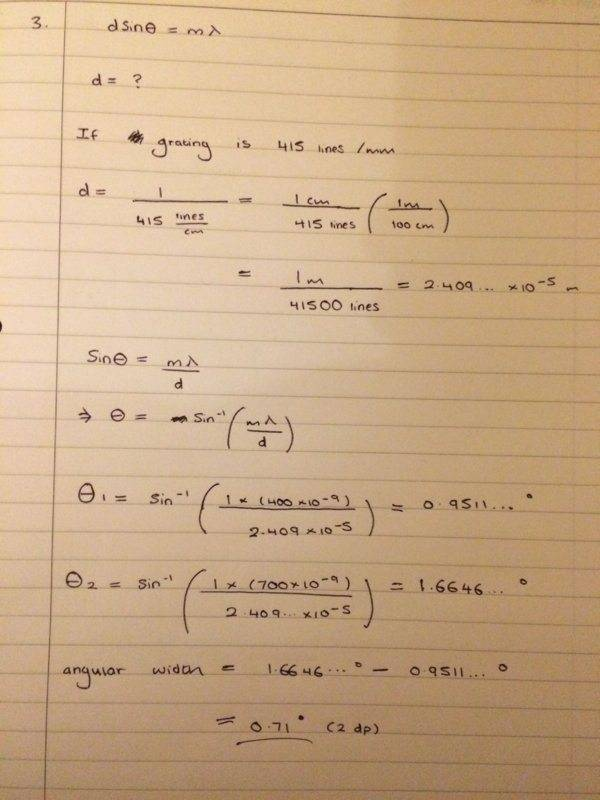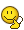# Angular width of first-order visible spectrum

Bolter
Homework Statement:
See image attached below
Relevant Equations:
dsin(theta) = m*lambda
This is a diffraction grating problem I have been given that I am trying to answerMade a attempt at it and just wanted to see if I done this correctly or not? I get an angular width of 0.71 degrees which is very smallAny help is much appreciated! Thanks

You switched from lines per millimetre to lines per centimetre at the start.

•Bolter
Bolter
You switched from lines per millimetre to lines per centimetre at the start.

Oh shoot yes you're rightI don't know what I was thinking there. This must change the whole answer then. I get my distance between adjacent slits to be 1/415,000 m = 2.409... x 10^-6 m

Running through the same process but with new d value now, I get the angular width to be 7.33 degrees now?